Next: A TYPOLOGY OF USES Up: APPLICATIONS OF CIRCUMSCRIPTION Previous: INTRODUCTION AND NEW DEFINITION

# A NEW VERSION OF CIRCUMSCRIPTION

Definition. Let A(P) be a formula of second order logic, where P is a tuple of some of the free predicate symbols in A(P). Let E(P,x) be a wff in which P and a tuple x of individual variables occur free. The circumscription of E(P,x) relative to A(P) is the formula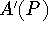defined by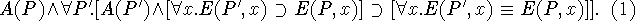[We are here writing A(P) instead of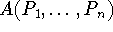for brevity and likewise writing E(P,x) instead of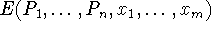]. Likewise the quantifier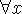stands for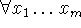. A(P) may have embedded quantifiers. Circumscription is a kind of minimization, and the predicate symbols in A(P) that are not in P itself act as parameters in this minimization. When we wish to mention these other predicates we write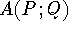and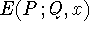where Q is a vector of predicate symbols which are not allowed to be varied.

There are two differences between this and (McCarthy 1980). First, in that paper E(P,x) had the specific form P(x). Here we speak of circumscribing a wff and call the method formula circumscription, while there we could speak of circumscribing a predicate. We still speak of circumscribing the predicate P when E(P,x) has the special form P(x). Formula circumscription is more symmetric in that any of the predicate symbols in P may be regarded as variables, and a wff is minimized; the earlier form distinguishes one of the predicates themselves for minimization. However, formula circumscription is reducible to predicate circumscription provided we allow as variables predicates besides the one being minimized.

Second, in definition (1) we use an explicit quantifier for the predicate variable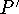whereas in (McCarthy 1980), the formula was a schema. One advantage of the present formalism is that nowis the same kind of formula as A(P) and can be used as part of the axiom for circumscribing some other wff.

In some of the literature, it has been supposed that nonmonotonic reasoning involves giving all predicates their minimum extension. This mistake has led to theorems about what reasoning cannot be done that are irrelevant to AI and database theory, because their premisses are too narrow.Next: A TYPOLOGY OF USES Up: APPLICATIONS OF CIRCUMSCRIPTION Previous: INTRODUCTION AND NEW DEFINITION

John McCarthy
Sat Jun 1 13:54:22 PDT 1996